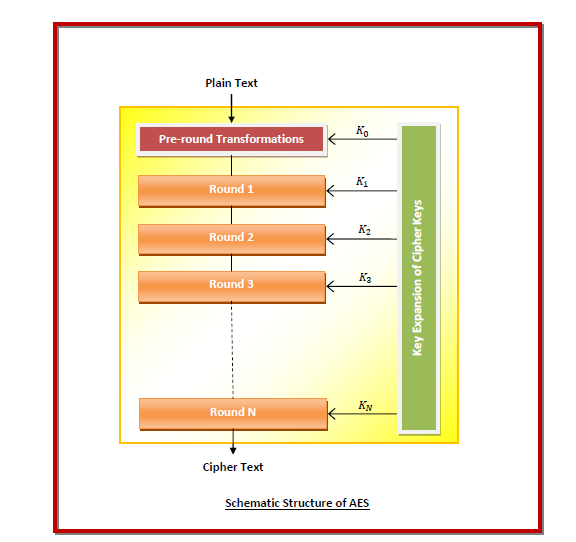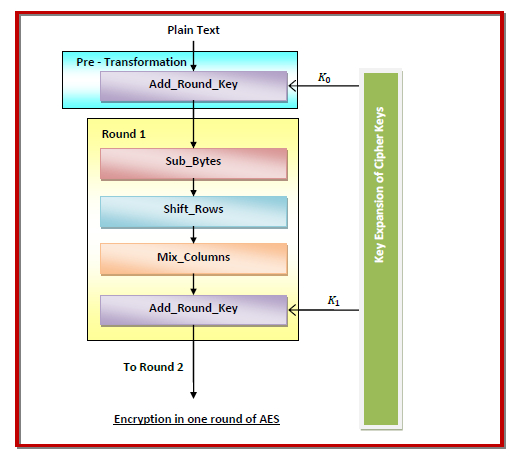The Advanced Encryption Standard, or AES, is an encryption standard established in 2001 by the National Institute of Standards and Technology (NIST) of USA. It is implemented worldwide both in hardware and software to encrypt sensitive data. AES finds wide usage while transmitting data over computer networks, particularly in wireless networks.

## Features of AES

• AES is a subset of Rijndael block cipher.
• It is a successor of Data Encryption Standard (DES) and is stronger and faster than DES.
• It is a symmetric key symmetric block cipher.
• It operates on 128-bit (16 bytes) data.
• The cipher key may be of 128, 192 or 256 bits.
• All computations are performed on bytes rather than bits.
• AES gives full specification and design details.
• It can be implemented using languages C and Java for software protection.

## Operation of AES

The encryption process of Advanced Encryption Standard is based upon substitution and permutation operations in iterative manner. The 16 bytes of data are arranged in a matrix of four columns and four rows. On this matrix, AES performs rounds of substitution-permutation operations. Each of these rounds uses a different cipher key, which is calculated from the original AES key. The number of rounds of operations depends upon the size of the key in the following manner −

• For 128-bit cipher key, 10 rounds
• For 192-bit cipher key, 12 rounds
• For 256-bit cipher key, 14 rounds

The following diagram shows the schematic structure −The encryption sub-processes in the above algorithm are −

• Key Expansion − The round keys are calculated from the cipher key using Rijndael's block cipher schedule.

• Pre–Transformation − This comprises of only 1 process namely Add_Round_Key. Here, XOR operation is performed on each data byte with a byte of the round key.

• Round 1 to Round (N-1) − Four sub-processes are performed here.

• Sub_Bytes − Non-linear substitution is performed on each byte whereby the byte is replaced with another byte as per a lookup table.

• Shift_Rows − Transposition is performed wherein a certain number of cyclical shifting of the last three rows is done.

• Mix_Columns − Mixing of rows and columns in a pre-defined manner is performed.

• Add_Round_Key − XOR operation is performed on each byte with a byte of the round key.

• Round N − The final round comprises of three sub-processes, namely −

• Sub_Bytes
• Shift_Rows